Homework Help Question & Answers

# Part A ConstantsI Periodic Table An ideal gas with γ = 1.4 occupies 6.0 L at...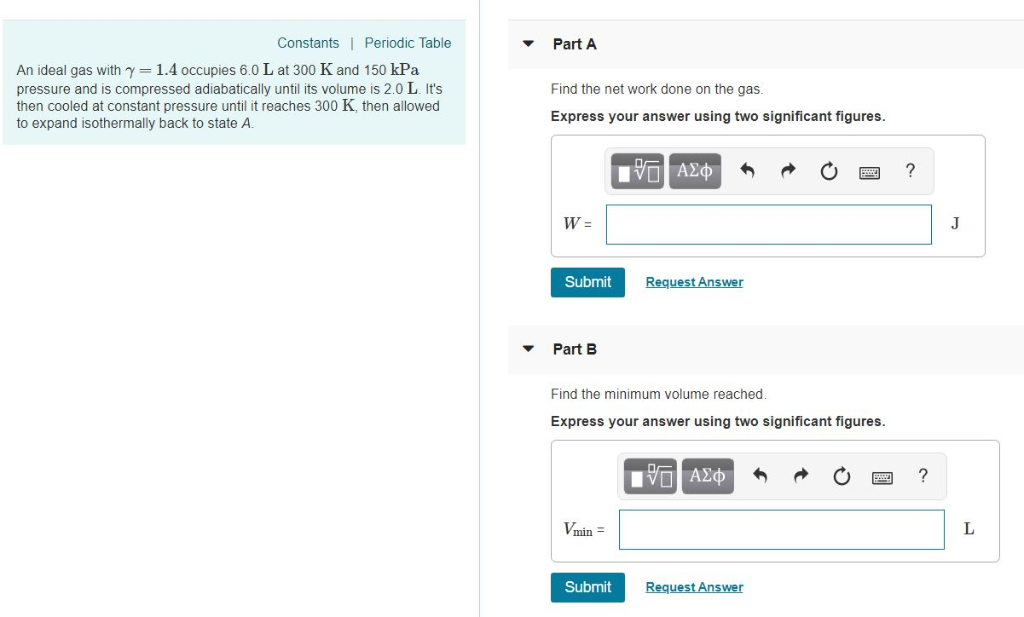Part A ConstantsI Periodic Table An ideal gas with γ = 1.4 occupies 6.0 L at 300 K and 150 kPa pressure and is compressed adiabatically until its volume is 2.0 L. It's then cooled at constant pressure until it reaches 300 K, then allowed to expand isothermally back to state A. Find the net work done on the gas Express your answer using two significant figures. 0図? W- Submit Request Answer Part B Find the minimum volume reached Express your answer using two significant figures. 0図? Vmin Submit Request Answer

#### Homework Answers

Answer #1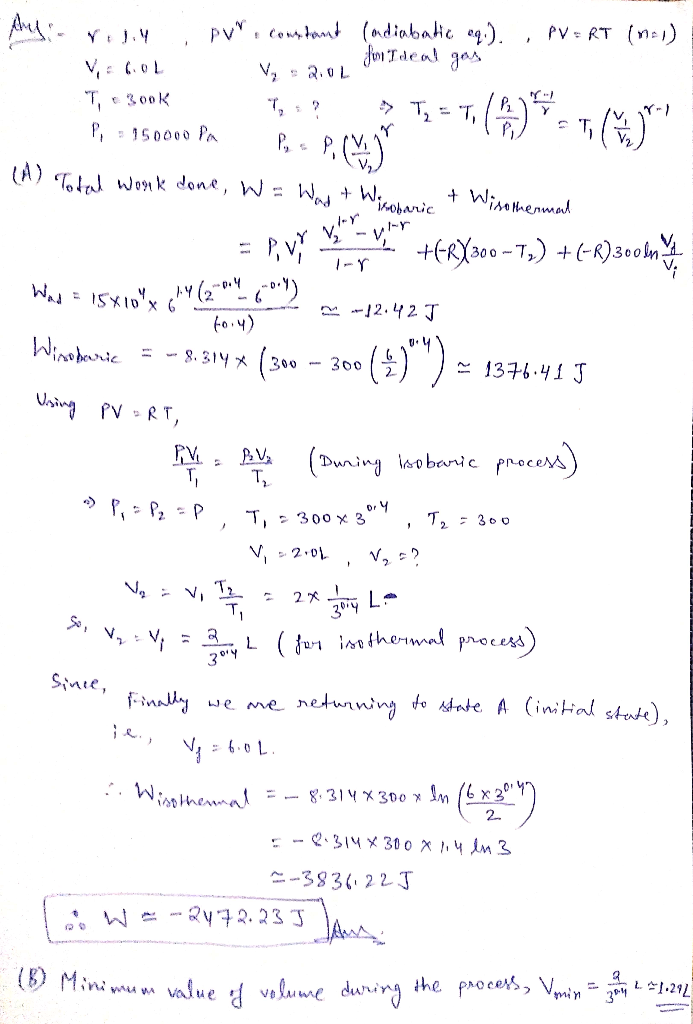Know the answer?
Your Answer:

#### Post as a guest

Your Name:

What's your source?

#### Earn Coin

Coins can be redeemed for fabulous gifts.

Not the answer you're looking for? Ask your own homework help question. Our experts will answer your question WITHIN MINUTES for Free.
Similar Homework Help Questions
• ### An ideal gas with ?=1.4 occupies 5.5L at 300 K and 150kPa pressure and is compressed...

An ideal gas with ?=1.4 occupies 5.5L at 300 K and 150kPa pressure and is compressed adiabatically until its volume is 2.0 L. It's then cooled at constant pressure until it reaches 300 K, then allowed to expand isothermally back to state A. A)Find the net work done on the gas B) Find the minimum volume reached.

• ### Part A Constants 1 Periodic Table Two cylinders each contain 0.30 mol of a diatomic gas...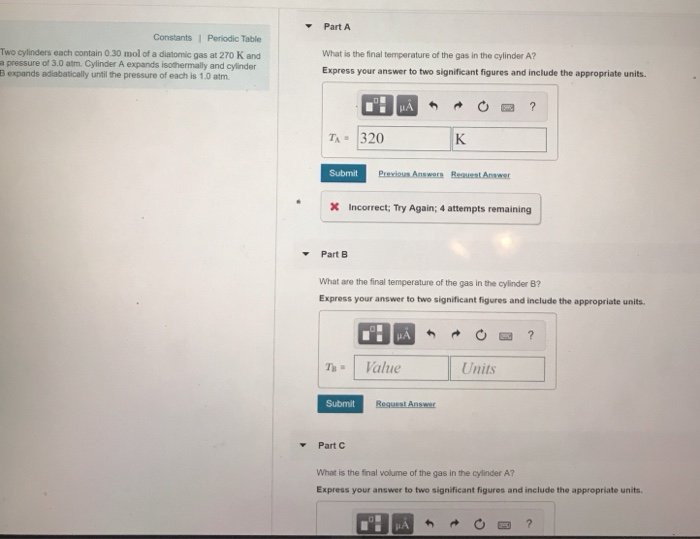Part A Constants 1 Periodic Table Two cylinders each contain 0.30 mol of a diatomic gas at 270 K and a pressure of 3.0 atm. Cylinder A expands isothermally and cylinder B expands adiabatically until the pressure of each is 1.0 atm What is the final temperature of the gas in the cylinder A? Express your answer to two significant figures and include the appropriate units. n 320 K Submit X Incorrect; Try Again; 4 attempts remaining Part B What...

• ### Problem 17.62 C 10 of Part A Constants 1 Periodic Table What is the final temperature...Problem 17.62 C 10 of Part A Constants 1 Periodic Table What is the final temperature of the gas in the cylinder A? Express your answer to two significant figures and include the appropriate units Two s each contain 0.10 mol of a diatomic gas at 350 K and a pressure of 3.0 atm. Cylinder A expands sothermally and cylinder B expands adiabatically unti the pressure of each is 1.0 atm TA Value Units Submit Request Answer -Part B What...

• ### Review Constants Periodic Table A0 12-mole sample of nitrogen gas occupies a volume of 205 L...Review Constants Periodic Table A0 12-mole sample of nitrogen gas occupies a volume of 205 L Part A What is the volume of 0 32 mol of nitrogen gas under the same conditions? Express your answer using two significant figures. VALDO ? Submit Request Answer Provide Feedback

• ### 10-9 Review | Constants Periodic Table Calculate each of the following quantities for an ideal gas....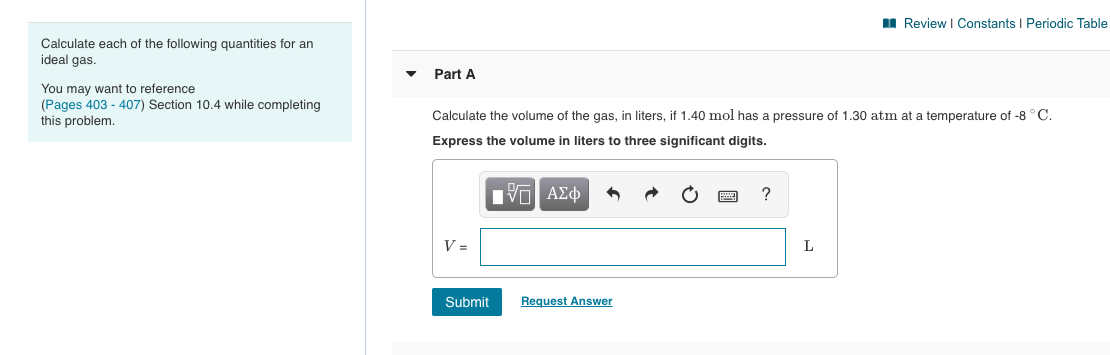10-9 Review | Constants Periodic Table Calculate each of the following quantities for an ideal gas. Part A You may want to reference (Pages 403 - 407) Section 10.4 while completing this problem. Calculate the volume of the gas, in liters, if 1.40 mol has a pressure of 1.30 atm at a temperature of -8°C. Express the volume in liters to three significant digits. O ACQ* * O O ? Submit Request Answer Part B Calculate the absolute temperature of...

• ### 1.06 mol of an ideal gas in a piston-cylinder initially occupies 9.0 L at 320 °C...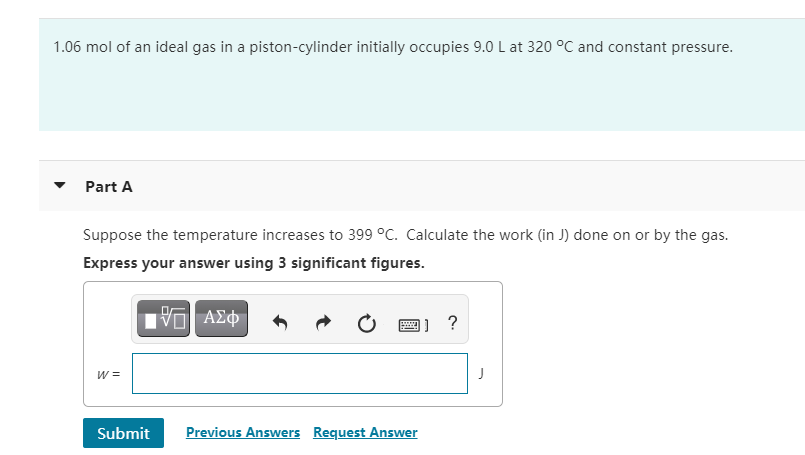1.06 mol of an ideal gas in a piston-cylinder initially occupies 9.0 L at 320 °C and constant pressure. Part A Suppose the temperature increases to 399 °C. Calculate the work in )) done on or by the gas. Express your answer using 3 significant figures. ΑΣΦ 2 ? w= Submit Previous Answers Request Answer

• ### Problem 18.65 Part A Review What is the final pressure of the gas? Express your answer...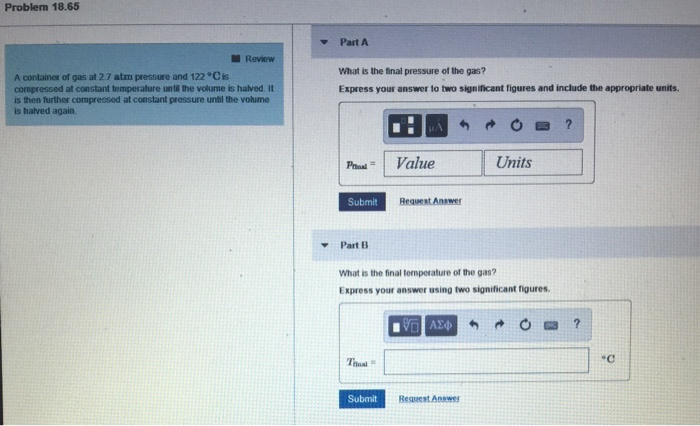Problem 18.65 Part A Review What is the final pressure of the gas? Express your answer to two significant figures and include the appropriate units A container of gas at 2.7 atm pressure and 122 Cis compressed at constant temperalture until the volume is halved. It is then further compressed at constant pressure until the volume is halved again P Value Units Submit Request Answer ▼Part13 What is the final temperature of the gas? Express your answer using two significant...

• ### A sample of a monatomic ideal gas occupies 5.00 L at atmospheric pressure and 300 K...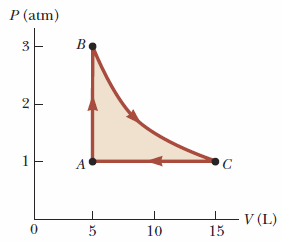A sample of a monatomic ideal gas occupies 5.00 L at atmospheric pressure and 300 K (point A in the figure below). It is warmed at constant volume to 3.00 atm (point B). Then it is allowed to expand isothermally to 1.00 atm (point C) and at last compressed isobarically to its original state. a. Find Q, W, and ΔEint for each of the processes. Q (kJ) W (kJ) Eint (kJ) A → B B → C C → A...

• ### Interested in doing part B 1. a) One mole of an ideal gas is compressed irreversibly...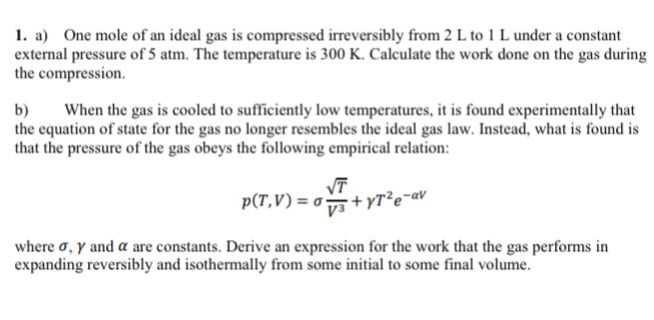Interested in doing part B 1. a) One mole of an ideal gas is compressed irreversibly from 2 L to 1 L under a constant external pressure of 5 atm. The temperature is 300 K. Calculate the work done on the gas during the compression. b When the gas is cooled to sufficiently low temperatures, it is found experimentally that the equation of state for the gas no longer resembles the ideal gas law. Instead, what is found is that...

• ### Part A Find the volume occupied by 20.0 g of helium gas at 20.0 °C and...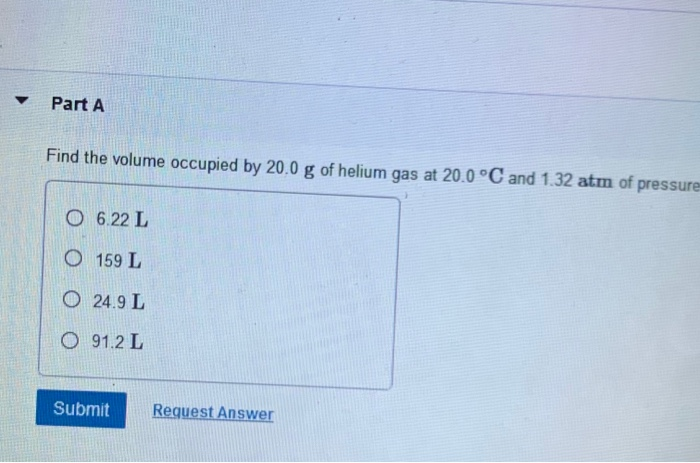Part A Find the volume occupied by 20.0 g of helium gas at 20.0 °C and 1.32 atm of pressure 0 6.22 L 0 159 L 0 24,9 L 0 91.2 L Submit Request Answer Part A Each gas sample has the same temperature and pressure. Which sample occupies the smallest volume? 0 20.0 g H, O 40.0 g Kr 40.0 g Ar O 4.0 g He Submit Request Answer Use Charles's law to complete the table. (Assume pressure and...